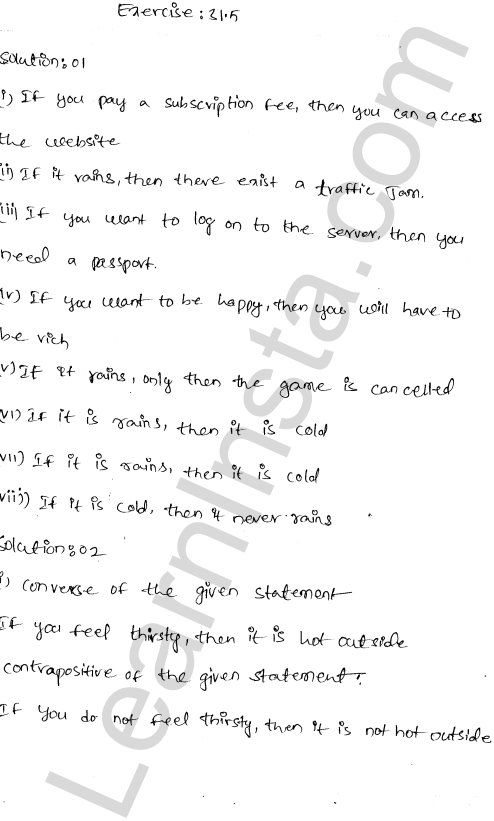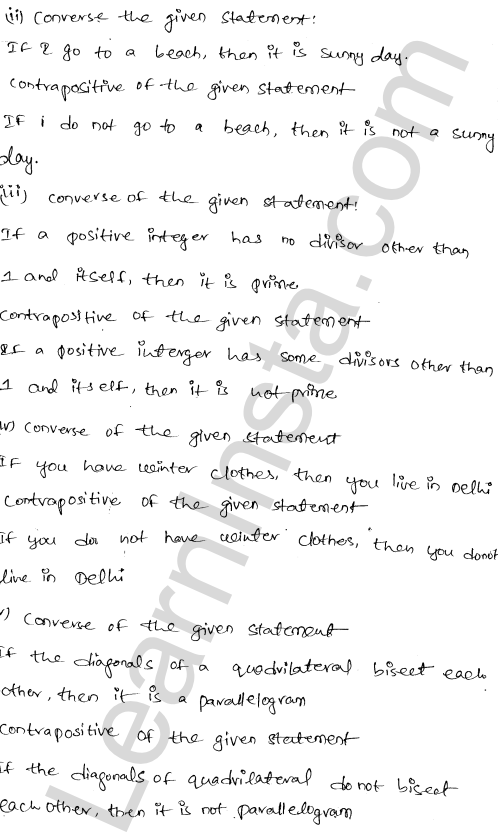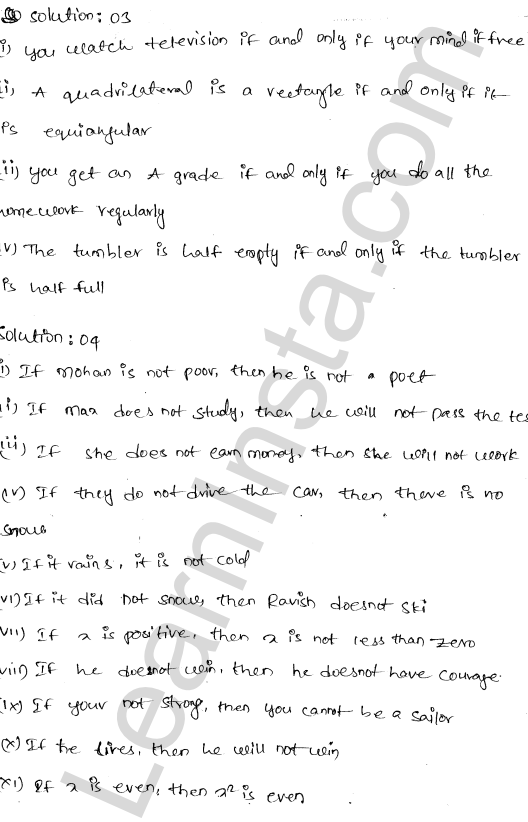# RD Sharma Class 11 Solutions Chapter 31 Mathematical Reasoning Ex 31.5

## RD Sharma Class 11 Solutions Chapter 31 Mathematical Reasoning Ex 31.5

These Solutions are part of RD Sharma Class 11 Solutions. Here we have given RD Sharma Class 11 Solutions 31 Mathematical Reasoning Ex 31.5.We hope the RD Sharma Class 11 Solutions Chapter 31 Mathematical Reasoning Ex 31.5, help you. If you have any query regarding RD Sharma Class 11 Solutions Chapter 31 Mathematical Reasoning Ex 31.5, drop a comment below and we will get back to you at the earliest.